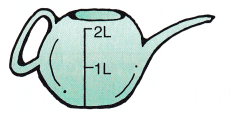Look at the clock. Write the time using numbers or words.

Question 1.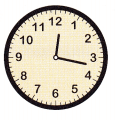__________ : ___________
Explanation:
The shorthand is on 12 and the minute hand is on 17. So, the time is 12:17
In words Twelve Seventeen.

Question 2.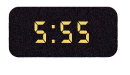5:55 in words is Five Fifty-Five or Five minutes to Six.

Question 3.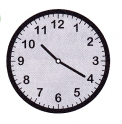__________ : ___________
The hour hand is on 10 and the minute hand is on 4. So, the time is 10:20.
In words Ten Twenty

Write how much time has passed

Question 4.
start 10:00
end 1:15
1:15 can be written in the 24-hour format as 13:15
To find how much time has passed we have to subtract 10 from 1:15
13 hour 15 mins
-10 hour 00 min
3 hour 15 mins
Thus the answer is 3 hours 15 mins.

Question 5.
start 6:40
end 3:00
3:00 can be written as 15:00
15 hour 00 mins
-6 hour 40 mins
8 hour 20 mins
Thus the answer is 8 hours 20 mins.

Question 6.
start 4:30
end 7:20
7 hour 20 mins
-4 hour 30 mins
2 hour 50 mins
Thus the answer is 2 hours 50 mins.

Question 7.
Cameron finishes his chores at 1:30. He spent 5 minutes watering houseplants, 30 minutes raking leaves, and 5 minutes making his bed. When did he start his chores? Use the number line and work backward to help you solve.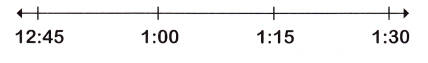Cameron finishes his chores at 1:30.
He spent 5 minutes watering houseplants, 30 minutes raking leaves, and 5 minutes making his bed.
5 + 30 + 5 = 40 minutes
We have to draw the number line backward.
Subtract 40 minutes from 1:30
1 hour 30 minutes – 40 minutes = 12:50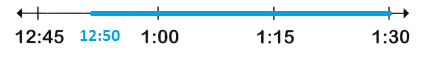Thus Cameron starts his chores at 12:50.

Place a check mark beside the better estimate for the amount each can hold.

Question 8.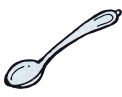___________ 3 L
___________ 5 mL
The spoon is small so it can hold 5 mL.
So, the estimated answer is 5mL.

Question 9.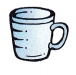___________ 30 L
___________ 300 mL
The cup can hold 300 mL
So, the estimated answer is 300 mL

Question 10.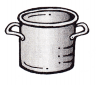___________ 100 mL
___________ 3 L
The container can hold 3 Liters.
So, the estimated answer is 3 L

Question 11.___________ 180 L
___________ 180 mL
The glass can hold 180 mL.
So, the estimated answer is 180 mL.

Place a check mark beside the better estimate of each object’s mass.

Question 12.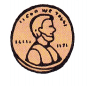___________ 3 g
___________ 3 kg
The estimated mass of the given object is 3 grams.

Question 13.___________ 100 kg
___________ 300 g
The estimated mass of the given object is 100 kg.

Question 14.___________ 170 g
___________ 2 kg
The estimated mass of the given object is 170 grams.

Question 15.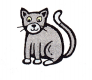___________ 4 g
___________ 4 kg
The estimated mass of the given object is 4 kg.

Question 16.
Find a heavy book or a container of laundry soap. Estimate how much the book or the laundry soap container weighs in pounds. If possible, check your estimate using a scale. Write the number of pounds.
Estimate: _______ lb
Measurement: ________ lb
The estimated weight of the laundry soap is about 3 lbs.
The measurement of the laundry soap is 3.5 lbs.

Place a check mark beside the better estimate of each object’s weight.

Question 17.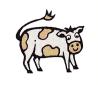___________ 900 lb
___________ 900 oz
The better estimate of the given object cow is 900 lbs.

Question 18.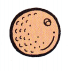___________ 4 lb
___________ 7 oz
The better estimate of the given object 7 ounces.

Question 19.___________ 35 lb
___________ 70 oz
The better estimate of the given object dog is 35 lbs.

Question 20.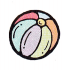___________ 10 lb
___________ 10 oz
The better estimate of the given object 10 oz.

Choose the correct operation and solve the word problem.

Question 21.
Mr. Hunter waters several large plants using this watering can. Each plant needs the amount of water in I full can. How many plants did Mr. Hunter water if he used 14 liters of water in all?